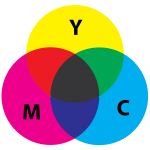# 浅谈调色

## 颜色模型

### RGB

RGB颜色模型常用于光学输出设备如屏幕和投影仪，它把光视为三种单色光混合而成：红色（Red）、绿色（Green）和蓝色（Blue）。

 R=0 B=0 B=0.25 B=0.5 B=0.75 B=1 G=0 G=0.25 G=0.5 G=0.75 G=1
 R=0.25 B=0 B=0.25 B=0.5 B=0.75 B=1 G=0 G=0.25 G=0.5 G=0.75 G=1
 R=0.5 B=0 B=0.25 B=0.5 B=0.75 B=1 G=0 G=0.25 G=0.5 G=0.75 G=1
 R=0.75 B=0 B=0.25 B=0.5 B=0.75 B=1 G=0 G=0.25 G=0.5 G=0.75 G=1
 R=1 B=0 B=0.25 B=0.5 B=0.75 B=1 G=0 G=0.25 G=0.5 G=0.75 G=1

### HSI、HSL和HSV

RGB颜色模型的一个缺点在于人并不擅长于在看到的颜色和其RGB值间转换，用RGB值挑选颜色并不直观。这样，与RGB等价的HSI、HSL和HSV就应运而生，它们仍然用三个分量，但这些分量有相对直观的意义：

• 色调（Hue）大致表示
• H含义为$H=60^{\circ}\begin{cases}\text{无定义}&,C=0\\ \frac{G-B}{C}&,M=R\\ \frac{B-R}{C}+2&,M=G\\ \frac{R-G}{C}+4&,M=B\end{cases}\mod 360^{\circ}$
• 饱和度（Saturation）大致表示颜色的深浅，如：
• HSI中的S含义为$S=1-\frac{m}{I}$
• HSL中的S含义为$S=\begin{cases}0&,V=0\\ \frac{C}{V}&,V\neq 0\end{cases}$
• HSV中的S含义为$S=\begin{cases}0&,L=1\\ \frac{C}{1-\vert 2L-1\vert}&,L\neq 1\end{cases}$
• 亮度（Lightness)大致表示颜色的明暗，如：
• HSI中的I含义为$I=\frac{R+G+B}{3}$
• HSL中的L含义为$L=\frac{M+m}{2}$
• HSV中的V含义为$V=M$
• 上面几种方案都把三种颜色地位平等的，但人眼却对不同色光敏感度不同，所以更合适的方案可能形如$0.299R+0.587G+0.114B$

 H=0 L=0 L=0.25 L=0.5 L=0.75 L=1 S=0 S=0.25 S=0.5 S=0.75 S=1
 H=30 L=0 L=0.25 L=0.5 L=0.75 L=1 S=0 S=0.25 S=0.5 S=0.75 S=1
 H=60 L=0 L=0.25 L=0.5 L=0.75 L=1 S=0 S=0.25 S=0.5 S=0.75 S=1
 H=90 L=0 L=0.25 L=0.5 L=0.75 L=1 S=0 S=0.25 S=0.5 S=0.75 S=1
 H=120 L=0 L=0.25 L=0.5 L=0.75 L=1 S=0 S=0.25 S=0.5 S=0.75 S=1
 H=150 L=0 L=0.25 L=0.5 L=0.75 L=1 S=0 S=0.25 S=0.5 S=0.75 S=1
 H=180 L=0 L=0.25 L=0.5 L=0.75 L=1 S=0 S=0.25 S=0.5 S=0.75 S=1
 H=210 L=0 L=0.25 L=0.5 L=0.75 L=1 S=0 S=0.25 S=0.5 S=0.75 S=1
 H=240 L=0 L=0.25 L=0.5 L=0.75 L=1 S=0 S=0.25 S=0.5 S=0.75 S=1
 H=270 L=0 L=0.25 L=0.5 L=0.75 L=1 S=0 S=0.25 S=0.5 S=0.75 S=1
 H=300 L=0 L=0.25 L=0.5 L=0.75 L=1 S=0 S=0.25 S=0.5 S=0.75 S=1
 H=330 L=0 L=0.25 L=0.5 L=0.75 L=1 S=0 S=0.25 S=0.5 S=0.75 S=1

### CMYK

CMYK颜色模型常用于彩色印刷，它使用四种颜料：青色（Cyan）、洋红（Magenta）、黄色（Yellow）和黑色（Key，原则上不是必须，但由于太常用和便宜，故也把它纳入）。由于印刷品本身不会发光，而只会反射外来的光，不同颜料由于不同的吸收/反射特性而使在入射光相同时反射光呈现不同颜色。值得注意的是，即使同一颜料对不同入射光也可呈现不同颜色，没有入射光时更是什么看起来都是黑色，所以一般假设入射光是白光。## 配色

1. 地铁线路图中通常用不同颜色区分线路
2. 图标中用不同颜色以便在受限的空间中区分简单对象
3. 网页常把导航和内容设成不同背景颜色，链接和非链接设成不同颜色
4. 应用程序常把不合法的数据标为红色

1. 如果要区分不同类型的东西，可以用不同的色调
• 要区分两类对象，可以用相反的两个色调；要区分三类对象，可以用相距$120^{\circ}$的三个色调
• 要区分两类对象，其中后一类又有两个子类，则可以用一个色调表示前一类，然后用与之相距$150^{\circ}$的两个色调表示两个子类
2. 如果要区分连续的多个程度（如温度、高度），可以使用不同的饱和度
3. 如果要区分不同状态的东西（如可用和暂停），可以用不同亮度

### 注意事项

1. 不宜使同时用过于接近的颜色，因为会人一种疑似调色错误的不一致感觉。
2. 不宜用颜色作为传递信息的惟一途径，因为色盲人士可能无法准确区分颜色，而视障辅助设备也往往不重视颜色。